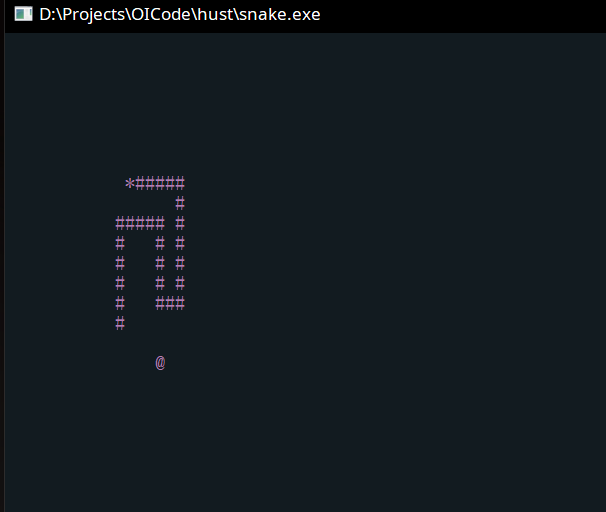book

flag
mode_edit### 输入

enum KeyRes { AUp = 0, ADown = 2, ALeft = 1, ARight = 3, AEsc = -1 };

using point = complex<int>;

map<KeyRes, point> d = {
{AUp, {-1, 0}}, {ADown, {1, 0}}, {ARight, {0, 1}}, {ALeft, {0, -1}}};

userPress = getKey();
if (d[userPress] + d[lastPress] == point({0, 0})) {
userPress = KeyRes(((int)userPress + 2) % 4);
}


### 输出

void moveTo(int x, int y) { cout << "\x1b[" << x << ';' << y << 'H'; }


void applyPatch(const vector<patch> &p) {
for (int i = 0; i < p.size(); ++i) {
moveTo(p[i].x + 1, p[i].y + 1);
cout << p[i].value;
cout.flush();
}
}